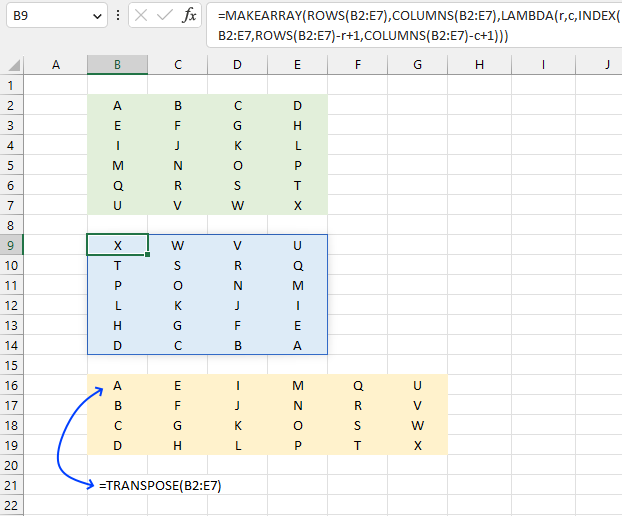Author: Oscar Cronquist Article last updated on March 17, 2023The MAKEARRAY function returns an array with a specific number of rows and columns calculated by applying a LAMBDA function.

## 1. MAKEARRAY Function Syntax

MAKEARRAY(rows, cols, lambda(row,col, calculation))

## 2. MAKEARRAY Function Arguments

 Argument Description rows The number of rows in the array to be created. Must be larger than 0 (zero). cols The number of columns in the array to be created. Must be larger than 0 (zero). row Required. A number representing the row in the array, the number changes from cell to cell. col Required. A number representing the column in the array, the number changes from cell to cell.

## 3. Flip/reverse a cell range both horizontally and verticallyThis example demonstrates how to flip or reverse values both horizontally and vertically using the MAKEARRAY function, see the blue cell range (B9:E14). The original source data is in a green cell range (B2:E7).

The third cell range colored yellow has values rearranged by the TRANSPOSE function in order to show the difference between transposing values and flip/reverse values.

Excel 365 formula in cell B9:

=MAKEARRAY(ROWS(B2:E7),COLUMNS(B2:E7),LAMBDA(r,c,INDEX(B2:E7,ROWS(B2:E7)-r+1,COLUMNS(B2:E7)-c+1)))

### Explaining formula

#### Step 1 - Count rows in the given cell range

The ROWS function calculate the number of rows in a cell range.

Function syntax: ROWS(array)

ROWS(B2:E7)-r+1

r is a variable, it starts from one and increments up to the number of rows in B2:E7. This makes the formula start from the bottom and not from the top.

#### Step 2 - Calculate rows in the given cell range

The COLUMNS function calculates the number of columns in a cell range.

Function syntax: COLUMNS(array)

COLUMNS(B2:E7)-c+1

#### Step 3 - Get value

The INDEX function returns a value or reference from a cell range or array, you specify which value based on a row and column number.

Function syntax: INDEX(array, [row_num], [column_num])

INDEX(B2:E7,ROWS(B2:E7)-r+1,COLUMNS(B2:E7)-c+1)

#### Step 4 - Build the LAMBDA function

The LAMBDA function build custom functions without VBA, macros or javascript.

Function syntax: LAMBDA([parameter1, parameter2, …,] calculation)

LAMBDA(r,c,INDEX(B2:E7,ROWS(B2:E7)-r+1,COLUMNS(B2:E7)-c+1))

#### Step 5 - Create and populate array

MAKEARRAY(ROWS(B2:E7),COLUMNS(B2:E7),LAMBDA(r,c,INDEX(B2:E7,ROWS(B2:E7)-r+1,COLUMNS(B2:E7)-c+1)))